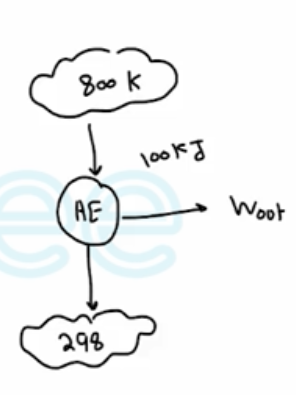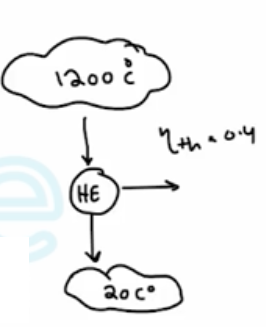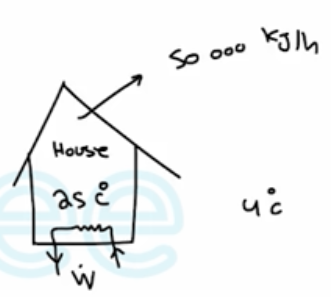Need Help?

Subscribe to Thermodynamics

###### \${selected_topic_name}
• Notes
• Comments & Questions

$\begin{array}{l}{\text { How much of the } 100 \mathrm{kJ} \text { of thermal energy at } 800 \mathrm{K} \text { can be converted to useful work? }} \\ {\text { Assume the environment to be at } 25^{\circ} \mathrm{C} .}\end{array}$$\eta_{th, rev}=1-\frac{T_0}{T_H}$

$=1-\frac{298}{800}=0.6275$

$W_{\text {max,out }}=W_{\text {rev,out }}=\eta_{\text {th,rev }}Q_{in}$

$=0.6275 * 100$

$=62.75 \mathrm{kJ}$

$\begin{array}{l}{\text { A heat engine that receives heat from a furnace and rejects waste heat to a river at } 20^{\circ} \mathrm{C}} \\ {\text { has a thermal efficiency of } 40 \text { percent. Determine the second-law efficiency of this power plant. }}\end{array}$

$\eta_{\mathrm{II}}=\frac{\eta_{th}}{\eta_{th \ rev}}$$\eta_{th, rev}=1-\frac{T_{0}}{T_{H}}=1-\frac{20+273}{1200+273}$

$=0.801$

$\eta_{II}=\frac{0.4}{0.801}=49.9 \%$

$* 100 \%$

$\begin{array}{l}{\text { A house that is losing heat at a rate of } 50,000 \mathrm{kJ} / \mathrm{h} \text { when the outside temperature drops to } 4^{\circ} \mathrm{C} \text { is }} \\ {\text { to be heated by electric r resistance heaters. If the house is to be maintained at } 25^{\circ} \mathrm{C} \text { at all times, }} \\ {\text { determine the reversible work input for this process and the irreversibility. }}\end{array}$$\dot{w}_{i n}=\dot{Q}_{out}=\dot{Q}_{H}$

$=\frac{50000 kJ/h}{3600} \longrightarrow 13.89 k w$

$\text { COP }_{HP, rev}=\frac{1}{1-T_L/T_H}$

$=\frac{1}{1-\frac{4+273}{25+273}}=14.2$

$\dot{W}_{\mathrm{rev \ in}}=$$\frac{\dot{Q}_{H}}{cop_{H P rev}}=\frac{13.89kw}{14.2}=0.978kw$

$\dot{I}=\dot{W}_{u \ i n}-\dot{W}_{rev \ i n}=13.89-0.978=12.91kw$

No comments yet